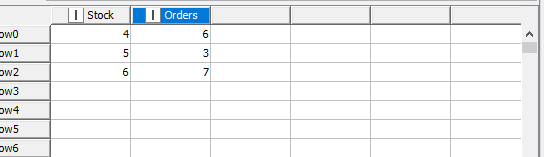# how to use recursive and chunk loop in the same time

Hello,
I want to design a loop that goes through row #1 and outputs a value that will be used in row #2. So it has to go through the rows one by one (not all at once) and bring back the data from the loop end node to the start node. So basically a combination of chunk and recursive loops. Is there a way to do this? Any ideas you may have are appreciated!

Hi @lamasewidan , could you share some data and show what the expected results for the first 3 lines, and of course explain how you got to these values?

This will help illustrate what you are trying to do, and there might be a more straight forward way to do this instead of using 2 loops nested in one another.

Thanks for the reply! Okay this is what I want to doThis is what the initial data would look like.
I want to look ONLY at row #1 and do the following: Stock-orders and the result would be identified by “gap” (maybe a new column named gap).
And then we move to row #2, if the gap of row#1 is >=0 subtract the orders of row1 from the stock of row 2 and this would be my new stock for row#2, else keep the stock of row#2 as it is
then calculate the gap for row#2 like we did for row#1, stock-orders (stock of row#2-orders of row#2 ofcourse)
and then we move to row#3
if the gap of row#2 is >=0 subtract the orders of row2 from the stock of row 3 and this would be my new stock for row#3, else keep the stock of row#3 as it is
then calculate the gap for row#3 like we did for row#2 and 1, stock-orders (stock of row#3-orders of row#3 ofcourse)
and then we keep doing the same thing if there are more rows.
I had 2 issues with doing this logic in knime; the first is going through every row individually which only the chunk loop does, the second is bringing back the gap of a row to be used in the math of the row after it and this can only be achieved by the recursive loop
thats why I initially asked how to used the 2 loops together. if there are other ways to do this please let me know!